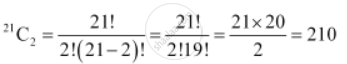Share

# How Many Chords Can Be Drawn Through 21 Points on a Circle? - Mathematics

#### Question

How many chords can be drawn through 21 points on a circle?

#### Solution

For drawing one chord on a circle, only 2 points are required.

To know the number of chords that can be drawn through the given 21 points on a circle, the number of combinations have to be counted.

Therefore, there will be as many chords as there are combinations of 21 points taken 2 at a time.

Thus, required number of chords  =Is there an error in this question or solution?